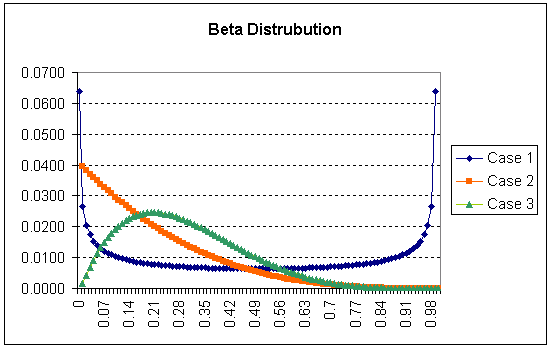# Using Beta Distribution for Estimating Recovery Rates

Most of the people use the beta distribution to model recovery rates.

In probability theory and statistics, the beta distribution is a family of continuous probability distributions defined on the interval (0, 1) parameterized by two positive shape parameters, typically denoted by α and β.

The function for the beta distribution is as follows:

$$f(x)=c.x^{a}(1-x)^{b}$$

c is the normalization constant.

We can input different values of α and β to obtain different shapes of recovery rates.

The mean and standard deviation of the dataset can be used as the two parameters α and β of the beta distribution using the BETADIST() function in excel. The following graph shows four shapes of the curves based on the different values of mean and standard deviation.This method is considered quite flexible because it only requires the mean and variance for calibration.

Download the following spreadsheet to see how the distribution is arrived at. This spreadsheet shows the estimation of recovery rates using beta distribution.